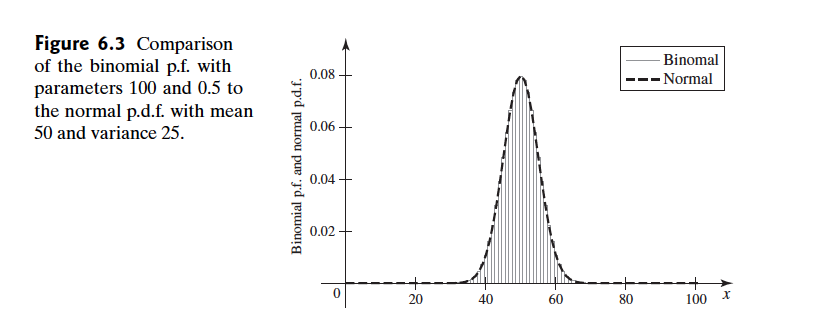Abstract: 本文介绍中心极限定理
Keywords: The Central Limit Theorem，The Normal distribution，The Delta Method

# 中心极限定理

## 中心极限定理 Statement of the TheoremPoisson分布的学习中，当我们遇到 $n$ 很大 $p$ 很小，而$np$ 有不大不小（这个标准有点含糊） 的时候可以用泊松分布来近似二项分布，上面的例子我们看出，当 $n$ 很大而 $p$ 又不太小的时候，正态分布可以用来近似二项分布。

Theorem Central Limit Theorem(Lindeberg and Levy) If the random variables $X_1,\dots,X_n$ form a random sample of size $n$ from a given distribution with mean $\mu$ and variance $\sigma^2$ ( $0<\sigma^2<\infty$ ) ,then for each fixed number $x$ ,
$$lim_{n\to \infty}Pr[\frac{\bar{X}_n-\mu}{\frac{\sigma}{n^{1/2}}}\leq x]=\Phi(x)$$
where $\Phi$ denotes the c.d.f. of the standard normal distribution.

1. $[0,1]$ 之间的均匀分布的期望是 $\frac{1}{2}$
2. 方差是 $\sigma^2=\frac{1}{12}$
3. 这里 $n=12$
4. $\bar{X}_n$ 根据中心极限定理，其分布近似为 $\mu_{\bar{X}_n}=\frac{1}{2},\sigma_{\bar{X}_n}^2=\frac{1}{144}$
5. 根据中心极限定理，设随机变量 $Z=12(\bar{X}_n-\frac{1}{2})$ 近似于标准正太分布，于是有：
\begin{aligned} Pr(|\bar{X}_n-\frac{1}{2}|\leq 0.1)&=Pr[12|\bar{X}_n-\frac{1}{2}|\leq 1.2]\\ &=Pr(|Z|\leq 1.2)\\ &\approx 2\Phi(1.2)-1\\ &=0.7698 \end{aligned}

Definition Convergence in Distribution/Asymptotic Distribution.Let $X_1,X_2,\dots$ be a sequence of random variables,and for $n=1,2,\dots$ ,let $F_n$ denote the c.d.f. of $X_n$ .Also,let $F’$ be a c.d.f. Then it is said that the sequence $X_1,X_2,\dots$ converges in distribution to $F’$ if
$$lim_{n\to \infty}F_n(x)=F’(x)$$
for all x at which $F’(x)$ is continuous.Sometimes,it is simply said that $X_n$ converges in distribution to $F’$ ,and $F’$ is called the asymptotic distribution of X_n,If $F’$ has a name ,then we say that X_n converges in distribution to that name

## Delta 方法 The Delta Method

🌰 ：

Theorem Delta Method. Let $Y_1,Y_2,\dots$ be a sequence of random variables,and let $F’$ be a continuous c.d.f. Let $\theta$ be a real number,and let $\alpha_1,\alpha_2,\dots$ be a sequence of positive numbers that increase to $\infty$ .Suppose that $a_n(Y_n-\theta)$ converges in distribution to $F’$ . Let $\alpha$ be a function with contimuous derivative such that $\alpha’(\theta)\neq 0$ .Then $a_n[\alpha(Y_n)-\alpha(\theta)]/\alpha’(\theta)$ converges in distribution to $F’$

1. $a_n\to\infty$ 的时候 $Y_n$ 会概率收敛到 $\theta$ 否则 $|a_n(Y_n-\theta)|\to \infty$ ，也就是不收敛。
2. 因为 $\alpha$ 是连续函数, $\alpha(Y_n)$ 将会非常接近 $\alpha(\theta)$
3. 使用泰勒级数(忽略更高阶项)：
$$\alpha(Y_n)\approx \alpha(\theta)+\alpha’(\theta)(Y_n-\theta)$$
4. 两边同时减去 $\alpha(\theta)$ 后乘以 $\frac{a_n}{\alpha’(\theta)}$ 得到：
$$\frac{a_n}{\alpha’(\theta)}(Y_n-\theta)\approx a_n(Y_n-\theta)$$

5. 4中结论左右两边都收敛到 $F’$

Corollary Delta Method for Average of a Ramdom Sample.Let $X_1,X_2\dots$ be a sequence of i.i.d. random variables from a distribution with mean $\mu$ and finite variance $\sigma^2$ .Let $\alpha$ be a function with continuous derivative such that $\alpha’(\mu)\neq 0$ .Then the asymptotic distribution of
$$\frac{n^{1/2}}{\sigma\alpha’(\mu)}[\alpha(\bar{X}_n)-\alpha(\mu)]$$
is the standard normal distribution.

0%